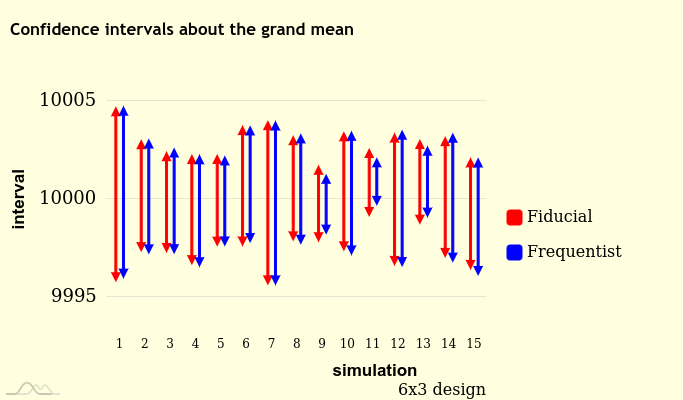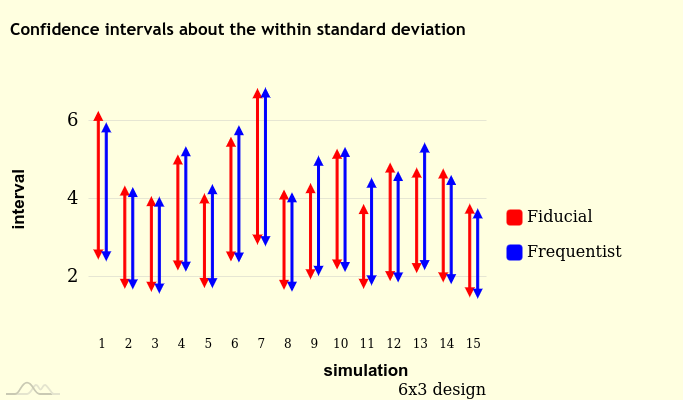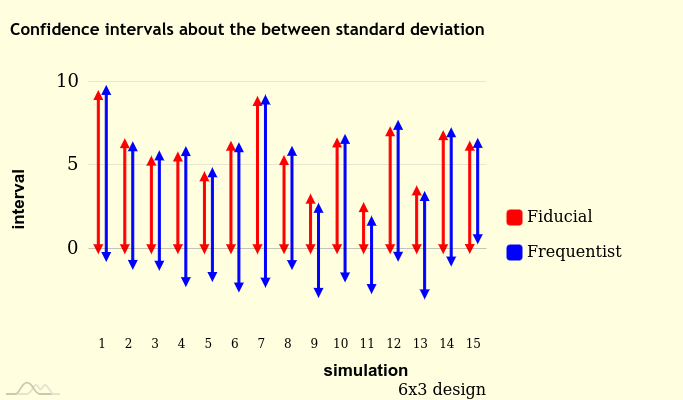The ‘gfilmm’ package

What does it do?

The ‘gfilmm’ package allows to generate simulations from the generalized fiducial distribution of the parameters of a Gaussian linear mixed model with categorical random effects (numeric random effects are not supported) and interval data. It also provides some helper functions to get summary statistics and confidence intervals.

The algorithm implemented in ‘gfilmm’ is the one described in the paper Generalized fiducial inference for normal linear mixed models written by Jessi Cisewski and Jan Hannig. It is coded in C++ and the code is based on the original Matlab code written by Jessi Cisewski.

Fiducial inference has something similar to Bayesian inference: the uncertainty about the parameters are represented by a distribution, the fiducial distribution, with the help of which we conduct inference on the parameters in a way similar to the Bayesian way, based on the posterior distribution of the parameters. The main difference is that there is no prior distribution (so fiducial inference is similar to objective Bayesian inference). The fiducial inference yields results close to the ones of the frequentist inference.

Note

Some pieces of code in the algorithm are run in parallel. In the examples below, as well as in the examples of the package documentation, I set nthreads = 2L because of CRAN restrictions: CRAN does not allow more than two threads and then it would not accept the package if a higher number of threads were set.

First example: a non-mixed linear model

The data must be given as a dataframe. Here we simulate data from a simple linear regression model:

set.seed(666L)
n <- 30L
x <- 1L:n
y <- rnorm(n, mean = x, sd = 2)
y_rounded <- round(y, digits = 1L)
dat <- data.frame(
ylwr = y_rounded - 0.05,
yupr = y_rounded + 0.05,
x = x
)

Now we run the fiducial sampler:

library(gfilmm)
fidSims <- gfilmm(
y = ~ cbind(ylwr, yupr), # interval data
fixed = ~ x,             # fixed effects
random = NULL,           # random effects
data = dat,              # data
N = 30000L,              # number of simulations
)

A summary of the fiducial simulations (the Pr(=0) column will be explained latter):

gfiSummary(fidSims)
#>                  mean    median        lwr      upr Pr(=0)
#> (Intercept) 0.8893567 0.8824914 -0.9514540 2.705711     NA
#> x           0.9098002 0.9103614  0.8060702 1.013240     NA
#> sigma_error 2.4438525 2.4051282  1.8897157 3.213041      0
#> attr(,"confidence level")
#>  0.95

The fiducial confidence intervals are close to the frequentist ones:

lmfit <- lm(y ~ x)
confint(lmfit)
#>                  2.5 %  97.5 %
#> (Intercept) -0.9589356 2.70406
#> x            0.8076886 1.01402

The fiducial cumulative distribution function of the slope:

Fslope <- gfiCDF(~ x, fidSims)
plot(Fslope, main = "Slope", ylab = expression("Pr("<="x)"))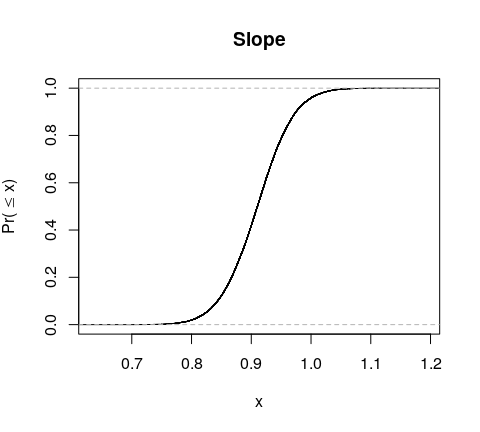To get a fiducial density, I recommend the ‘kde1d’ package:

library(kde1d)
kfit <- kde1d(fidSims$VERTEX[["x"]], weights = fidSims$WEIGHT, mult = 4)
curve(dkde1d(x, kfit), from = 0.7, to = 1.1, col = "red", lwd = 2)
# observe the resemblance with the distribution of the
# frequentist estimate of the slope:
curve(
dnorm(x, coef(lmfit)["x"], sqrt(vcov(lmfit)["x","x"])), add = TRUE,
col = "blue", lwd = 2, lty = "dashed"
)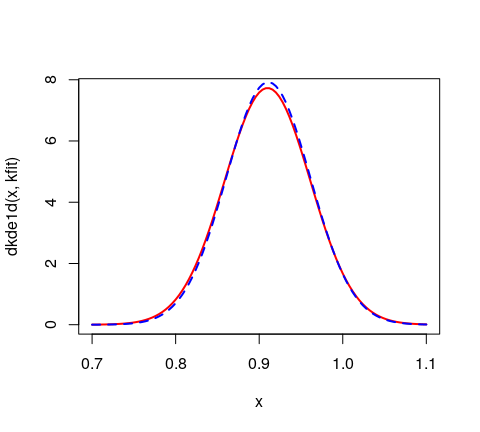The fiducial simulations are weighted, so it makes no sense to plot them without taking the weights into account. We can get “pseudo-simulations” of the fiducial distribution by picking the fiducial simulations at random according to their weight:

indcs <- sample.int(30000L, replace = TRUE, prob = fidSims$WEIGHT) pseudoSims <- fidSims$VERTEX[indcs,]
library(GGally)
#> Registered S3 method overwritten by 'GGally':
#>   method from
#>   +.gg   ggplot2
ggpairs(
pseudoSims,
upper = list(continuous = ggally_density),
lower = list(continuous = wrap("points", alpha = 0.1))
)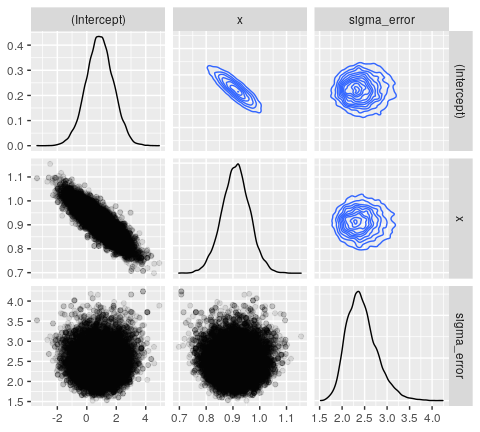The scatterplot of the intercept and the slope has the same shape as the frequentist confidence ellipse of these two parameters:

library(car)
dataEllipse(
pseudoSims[["(Intercept)"]], pseudoSims[["x"]],
levels = c(0.5,0.95), col = c("black", "red"),
xlab = "Intercept", ylab = "Slope"
)
confidenceEllipse(
lmfit, 1:2, levels = c(0.5,0.95), add = TRUE,
col = "blue", lty = "dashed"
)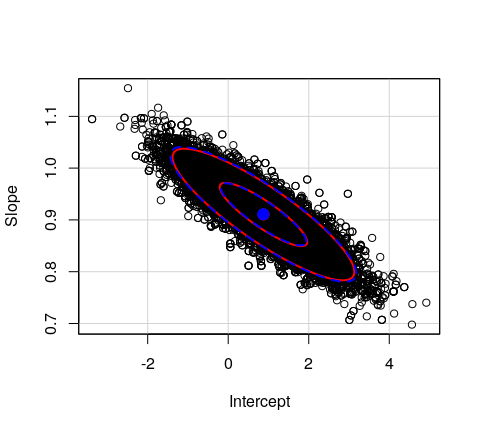Fiducial predictive inference.

The gfilmmPredictive function samples the generalized fiducial predictive distribution. All the functions seen above can be applied to the output.

fpd <- gfilmmPredictive(fidSims, newdata = data.frame(x = c(1, 30)))
gfiSummary(fpd)
#>         mean    median       lwr       upr
#> y1  1.785845  1.792856 -3.391241  6.988559
#> y2 28.166701 28.161277 22.974565 33.315927
#> attr(,"confidence level")
#>  0.95

Compare with the frequentist approach:

predict(lmfit, newdata = data.frame(x = c(1, 30)), interval = "prediction")
#>         fit       lwr       upr
#> 1  1.783417 -3.408471  6.975305
#> 2 28.198198 23.006310 33.390086

A mixed model

Now let us simulate some data from a one-way ANOVA model with a random factor:

mu           <- 10000 # grand mean
sigmaBetween <- 2
sigmaWithin  <- 3
I            <- 6L # number of groups
J            <- 5L # sample size per group

set.seed(31415926L)
groupmeans <- rnorm(I, mu, sigmaBetween)
y          <- c(
vapply(groupmeans, function(gmean) rnorm(J, gmean, sigmaWithin), numeric(J))
)
y_rounded  <- round(y, digits = 1L)
dat        <- data.frame(
ylwr = y_rounded - 0.05,
yupr = y_rounded + 0.05,
group = gl(J, I)
)

We run the fiducial sampler:

fidSims <- gfilmm(
~ cbind(ylwr, yupr), ~ 1, ~ group, data = dat, N = 30000L, nthreads = 2L
)

Observe that the between standard deviation sigma_group has a positive value in the Pr(=0) column:

gfiSummary(fidSims)
#>                    mean      median         lwr          upr     Pr(=0)
#> (Intercept) 9999.982067 9999.971777 9997.482236 10002.448921         NA
#> sigma_group    2.064531    1.787802    0.000000     5.610552 0.03191752
#> sigma_error    2.571030    2.519884    1.899821     3.519749 0.00000000
#> attr(,"confidence level")
#>  0.95

What does it mean? The fiducial distributions of the variance components have a mass at zero, and this value is the probability that the between standard deviation equals zero. So you have to be careful if you are interested in the fiducial density of a standard deviation: if Pr(=0) is not null for the standard deviation you are interested in, the fiducial distribution of this standard deviation does not have a density. It has a mass at zero, and a density on the strictly positive real numbers.

Compare the fiducial confidence interval of the grand mean to its Kenward-Roger confidence interval:

library(lmerTest)
library(emmeans)
fit <- lmer(y ~ (1|group), data = dat)
emmeans(fit, ~ 1)
#>  1       emmean      SE df lower.CL upper.CL
#>  overall  10000 0.83263  4   9997.7    10002
#>
#> Degrees-of-freedom method: kenward-roger
#> Confidence level used: 0.95

Actually the design is balanced, and we can get the same confidence interval by the frequentist method:

library(AOV1R)
aovfit <- aov1r(y ~ dat\$group)
confint(aovfit)
#>               estimate         lwr          upr
#> grandMean 10000.000191 9997.688438 10002.311943
#> within        2.575337    2.019727     3.555018
#> between       1.536546   -0.314190     5.239974
#> total         2.998889    2.432479     5.885821
#>
#> attr(,"confidence level")
#>  0.95
#> attr(,"standard deviations")
#>  TRUE

With gfiConfInt we can get a fiducial confidence interval for any parameter of interest, for example the coefficient of total variation:

gfiConfInt(~ sqrt(sigma_group^2 + sigma_error^2)/(Intercept), fidSims)
#>         2.5%        97.5%
#> 0.0002326897 0.0006209810

How many fiducial simulations should we run?

This is a good question. Unfortunately I have no clue about the answer. A good practice consists in running several fiducial samplers and to check whether the results change. Since we have already tried 30000 simulations, let us try with 40000 and 50000:

gfs <- lapply(c(40000L, 50000L), function(N){
gfilmm(~ cbind(ylwr, yupr), ~ 1, ~ group, data = dat, N = N, nthreads = 2L)
})
lapply(gfs, gfiSummary)
#> []
#>                    mean      median         lwr          upr     Pr(=0)
#> (Intercept) 9999.965740 9999.963689 9997.576113 10002.334580         NA
#> sigma_group    1.977488    1.726288    0.000000     5.400081 0.03936177
#> sigma_error    2.591382    2.540507    1.908865     3.579878 0.00000000
#> attr(,"confidence level")
#>  0.95
#>
#> []
#>                    mean      median         lwr          upr     Pr(=0)
#> (Intercept) 9999.959181 9999.955233 9997.547636 10002.389300         NA
#> sigma_group    2.025507    1.758480    0.000000     5.510785 0.03502531
#> sigma_error    2.577722    2.529386    1.898784     3.543214 0.00000000
#> attr(,"confidence level")
#>  0.95

We find similar results, so N = 30000L should be enough.

Note

Never combine the fiducial simulations of two different runs. That makes no sense.

A small simulation study

I have performed some simulations to evaluate the frequentist coverage of the fiducial 95%-confidence intervals for two different designs of a one-way ANOVA model with a random factor: the first one with three groups and two replicates per group (3x2 design), and the second one with six groups and three replicates per group (6x3 design). I compared with the frequentist confidence intervals calculated by the ‘AOV1R’ package. These ones are theoretically exact, except the one about the between standard deviation.

3x2 design

Here are the coverage probabilities of the frequentist confidence intervals:

Interval
Parameter   two-sided left-sided right-sided
grandMean    95.250     97.800       97.45
within       94.600     97.550       97.05
between      94.575     96.975       97.60

And the ones of the fiducial confidence intervals:

Interval
Parameter   two-sided left-sided right-sided
grandMean    99.450     99.750     99.700
within       95.800     97.950     97.850
between      99.425     99.975     99.450
total        96.850     99.925     96.925
CV           96.850     99.925     96.925

The figures below show the confidence intervals for fifteen simulations.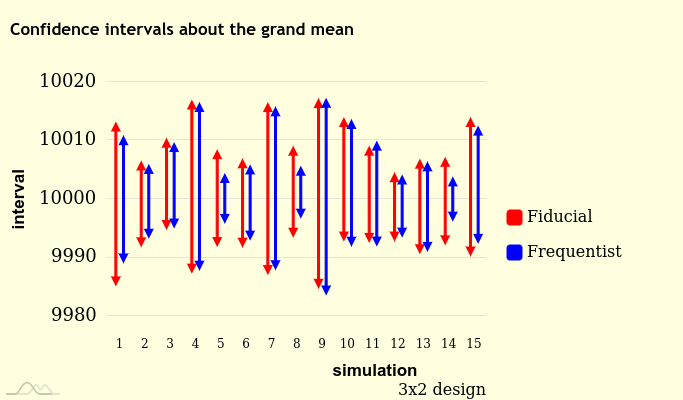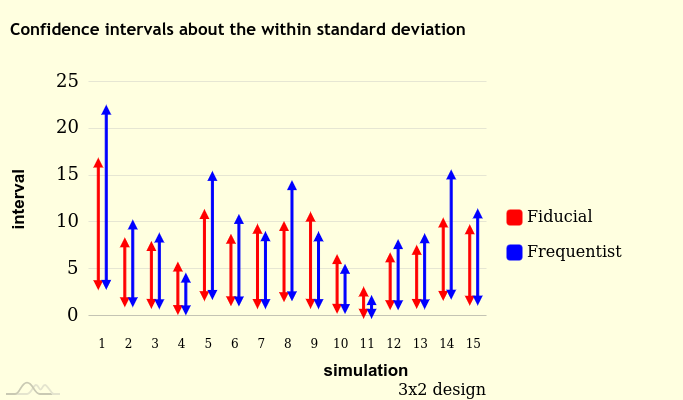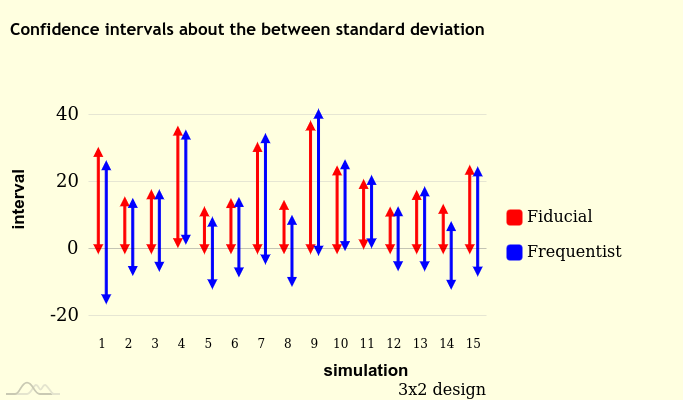6x3 design

Coverage probabilities of the frequentist confidence intervals:

Interval
Parameter   two-sided left-sided right-sided
grandMean     95.10     97.400      97.700
within        94.85     97.450      97.400
between       94.95     97.275      97.675

Coverage probabilities of the fiducial confidence intervals:

Interval
Parameter   two-sided left-sided right-sided
grandMean    96.925     98.400      98.525
within       95.475     97.925      97.550
between      98.325     99.675      98.650
total        95.950     99.375      96.575
CV           95.975     99.375      96.600

Confidence intervals for fifteen simulations: